JEE  >  Test: Geometric Progressions

# Test: Geometric Progressions

Test Description

## 10 Questions MCQ Test Mathematics (Maths) Class 11 | Test: Geometric Progressions

Test: Geometric Progressions for JEE 2022 is part of Mathematics (Maths) Class 11 preparation. The Test: Geometric Progressions questions and answers have been prepared according to the JEE exam syllabus.The Test: Geometric Progressions MCQs are made for JEE 2022 Exam. Find important definitions, questions, notes, meanings, examples, exercises, MCQs and online tests for Test: Geometric Progressions below.
Solutions of Test: Geometric Progressions questions in English are available as part of our Mathematics (Maths) Class 11 for JEE & Test: Geometric Progressions solutions in Hindi for Mathematics (Maths) Class 11 course. Download more important topics, notes, lectures and mock test series for JEE Exam by signing up for free. Attempt Test: Geometric Progressions | 10 questions in 10 minutes | Mock test for JEE preparation | Free important questions MCQ to study Mathematics (Maths) Class 11 for JEE Exam | Download free PDF with solutions
 1 Crore+ students have signed up on EduRev. Have you?
Test: Geometric Progressions - Question 1

### For a G.P. the ratio of the 7th and the third terms is 16. The sum of 9 terms is 2555. What is the first term?

Detailed Solution for Test: Geometric Progressions - Question 1

ar6/ar2 = 16
r4 = 16
r4 = (2)4
r = 2
S9 = a(rn - 1)
2555 = a((2)9 - 1)
2555 = a(512-1)
2555/511 = a
a = 5

Test: Geometric Progressions - Question 2

### The G.M. of 5 and 8 is

Detailed Solution for Test: Geometric Progressions - Question 2

The geometric mean of two numbers, say x, and y is the square root of their product x * y.
Mean = [x * y]1/2
= [5 * 8]1/2
= 1/2
= 2(10)1/2

Test: Geometric Progressions - Question 3

### The 10th term of an G.P. is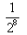and the 5th term is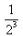.What is the Common Ratio?

Detailed Solution for Test: Geometric Progressions - Question 3

ar9 = 1/(2)8 -----------------------(1)
ar3 = 1/(2)2 ------------------------------(2)
Comparing eq (1) & (2)
1/(2)8 r9 = 1/(2)^2 r4
1/r5 = (2)5
r5 = 1/(2)5
r = 1/2

Test: Geometric Progressions - Question 4

A sequence a1, a2, a3,…, an is called ______ progression, if each term is non-zero  and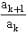= constant for

Detailed Solution for Test: Geometric Progressions - Question 4

The expression depicts the ratio of two numbers, if the ratio between the numbers is constant, then it will definately form a GP.

Test: Geometric Progressions - Question 5

Which of the following sequeces in GP will have common ratio 3,where n is an Integer?

Detailed Solution for Test: Geometric Progressions - Question 5

gn = 6( 3n-1) it is a geometric expression with coefficient of constant as 3n-1.So it is GP with common ratio 3.

Test: Geometric Progressions - Question 6

The third term of a G.P. is 3, the product of first five terms of this progression is:

Detailed Solution for Test: Geometric Progressions - Question 6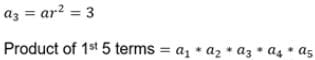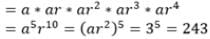Test: Geometric Progressions - Question 7

The sum of n terms of the sequence 8, 88, 888,…. is:

Detailed Solution for Test: Geometric Progressions - Question 7

8(1) + 8(11) + 8(111) + 8(1111) +........up to n times
= 8{ 1 + 11 + 111 + 1111 + ....... up to n times}
= 8/9{9 + 99 + 999 + 9999 + ..... up to n times}
= 8/9 {(10-1 )+ (100-1) + (1000-1) + (10000-1) + ........up to n times}
= 8/9 {(10 + 100 + 1000 + 10000 +...... n times) - (n×1)}
= 8/9{( (10×(10n -1))/(10-1)) -n}
= 80/81(10n - 1) - n
=  8/81(10n+1 - 10 -9n)

Test: Geometric Progressions - Question 8

Which term of the following sequence is 64 ?
2 , 2√2, 4, .....

Detailed Solution for Test: Geometric Progressions - Question 8

Given sequence : 2, 2√2, 4….
First term a1 = a = 2 and 2nd term a2 = 2√2, then
Common ratio r = a2/a = (2√2)/2
Let an = 64
∴ ar(n-1) = 64
⇒ 2.(√2)(n-1) = 32
⇒ (2)(n-1)/2 = 32
∴ (2)(n-1)/2 = (2)5
⇒ (n − 1)/2 = 5,
⇒ n = 11

Test: Geometric Progressions - Question 9

How many terms of the G.P. 4 + 16 + 64 + … will make the sum 5460?

Detailed Solution for Test: Geometric Progressions - Question 9

Sum (Sn) = a x (rn -1)/(r-1)
5460 = 4 x (4n -1)/3
16380 = 4n+1 - 4

16384 = 4n+1

4= 4n+1

7 = n + 1

n = 6

Test: Geometric Progressions - Question 10

What is the 50th term of the sequence √3, 3, 3√3, 9, ......

Detailed Solution for Test: Geometric Progressions - Question 10

an = ar(n-1)
Given, a = √3, r = 3/√3
​r = √3
a50 = ar(n-1)
= (√3)(√3)(50-1)
= (√3)(√3)49
= (√3)50

## Mathematics (Maths) Class 11

156 videos|171 docs|132 tests
 Use Code STAYHOME200 and get INR 200 additional OFF Use Coupon Code
Information about Test: Geometric Progressions Page
In this test you can find the Exam questions for Test: Geometric Progressions solved & explained in the simplest way possible. Besides giving Questions and answers for Test: Geometric Progressions, EduRev gives you an ample number of Online tests for practice

## Mathematics (Maths) Class 11

156 videos|171 docs|132 tests